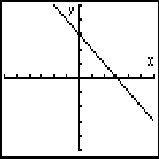# Linear Equation Test

 1) Find the gradient (slope) of this line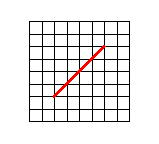4 1 -1 -2 2) Find the gradient (slope) of this line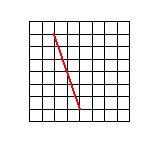3 1 -3 2 -2 -1 3) What value is the y intercept of this line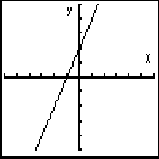4 2 3 1 4) Find the equation of this line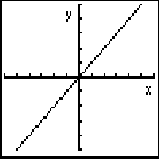y=2x y=3 y=2x + 3 y=x 5) Find the equation of this line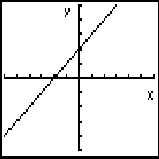y=x + 5 y=2x + 4 y=x + 2 y=5 - 2x 6) Which graph shows the line y = 2x + 4 Graph 1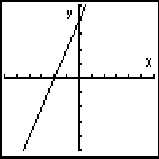Graph 2Graph 3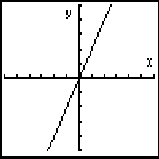7) Which graph shows the line y = 3 - x Graph 1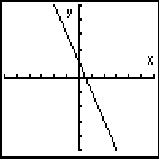Graph 2Graph 3